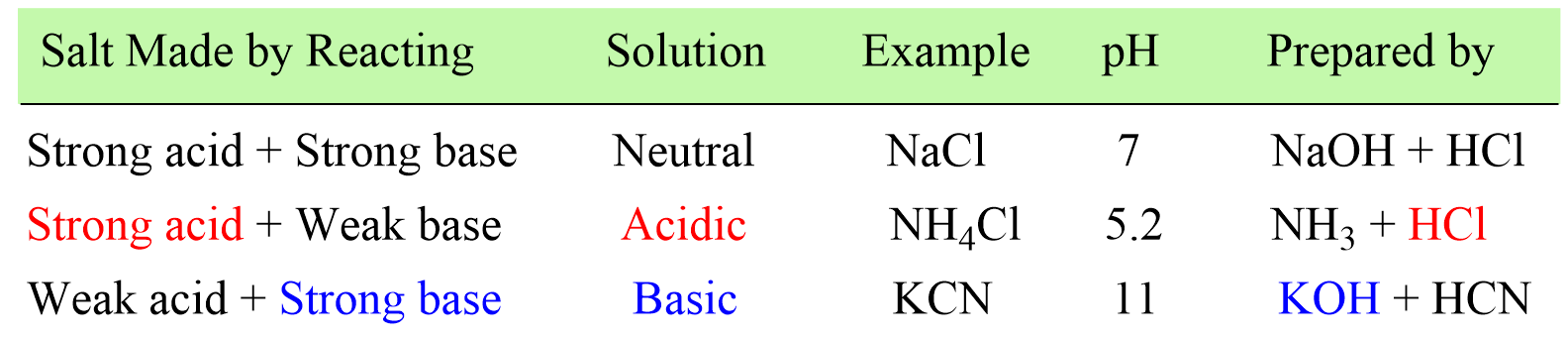## General Chemistry

In the previous post couple of posts, we learned how to determine whether the solution of a salt is going to be acidic or basic, and calculate the pH of a salt solution.

In short, if the salt is made of strong acid and a weak base, then it will give an acidic solution. If it is prepared from a strong base and weak aid, the solution will be basic, and if it is a combination of a strong acid and a strong base, a neutral solution will be formed.Now, what if the salt contains a cation of a strong base and an anion of a strong acid. With this combination, the salt contains an acidic cation and a basic anion, and the question is whether the solution is going to be acidic or basic.

# When Ka < Kb, a Basic Solution is Formed

For example, ammonium carbonate (NH4)2CO3 contains a cation of a weak base ammonia (NH3) and an anion of a weak acid H2CO3.

Remember, when determining the pH of a salt solution, we identified the cation of the weak component and reacted it with water to see if it produces H3O+ or OH ions. And now, because both ions are from a weak acid and base, we need to react them with water and see which one produces more H3O+ or OH.

NH4+(aq) + H2O(l) → NH3(aq) + H3O+(aq) (makes acidic)

CO32-(aq) + H2O(l) → HCO3(aq) + OH(aq) (makes basic)

If the hydrolysis of NH4+ produces more H3O+ than CO32- produces OH, the solution will be acidic. And vice versa, if there is more OH- produced in the solution, it will become a basic solution.

So, how do we know which of the ions will be produced in a greater quantity?

This is determined based on the values of Ka and Kb.

NH4+(aq) + H2O(l) → NH3(aq) + H3O+(aq) Ka = 5.6 x 10-10

CO32-(aq) + H2O(l) → HCO3(aq) + OH(aq) Kb = 1.8 x 10-4

The Kb of carbonate ion is larger than the Ka of ammonia, and therefore, the solution will be basic because there is more OH- than H3O+ produced in the solution.

# When Ka > Kb, an Acidic Solution is Formed

Let’s also consider an example of a salt that yields an acidic solution.

For example, The NH4F is salt of a weak base ammonia and a weak acid HF. To determine whether the solution is going to be basic or acidic, we write the reaction of the cation and the anion with water:

NH4+(aq) + H2O(l) → NH3(aq) + H3O+(aq) Ka = 5.6 x 10-10

F(aq) + H2O(l) → HF(aq) + OH(aq) Kb = 2.9 x 10-11

Comparing the values of Ka for the acidic cation and Kb for the basic anion, we determine the solution will be acidic because Ka > Kb.

# When Ka ≈ Kb, a Neutral Solution is Formed

One more possibility is when the salt of a weak acid and weak base produces a neutral solution. This is somewhat similar to what we saw for salts containing strong acid and base components. However, the difference is that in this case, there is a reaction with water, but because Ka Kb, the hydronium and hydroxide ions are formed in approximately equal quantities and the solution stays neutral.

In summary, remember these patterns of the Ka and Kb relationship when determining the acidity of salt solution.

• Ka > Kb: If Ka for the cation is greater than Kb for the anion, the first reaction will produce more H3O+ and therefore, pH < 7.
• Ka < Kb: If Ka for the cation is less than Kb for the anion, the second reaction will produce more OH and therefore, pH > 7.
• Ka Kb: If Ka for the cation and Kb for the anion are about equal, the concentrations of H3O+ and OH ions will be balanced, so the pH ≈ 0.

Check Also